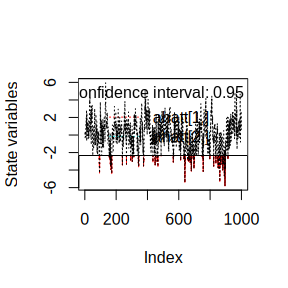# Fast Kalman Filter

To demonstrate the use of the FKF package this example shows how to fit an ARMA(2, 1) model using this Kalman filter implimentation and teh extraction of the resulting filtered and smoothed estimates.

library('FKF')

# Generating synthetic data

A data set of 1000 points is generated from the model $a_{t} = \frac{-0.2}{1 - 0.6z^{-1} - 0.2z^{-1}}\eta_t$ where $$\eta_{t} \sim N\left(0,0.2\right)$$

n <- 1000

## Set the AR parameters
ar1 <- 0.6
ar2 <- 0.2
ma1 <- -0.2
sigma <- sqrt(2)

a <- arima.sim(model = list(ar = c(ar1, ar2), ma = ma1), n = n,
innov = rnorm(n) * sigma)

# Estimation

To estimate the parameters using numerical optimisation two functions are specified. The first creates a state space representation out of the four ARMA parameters

arma21ss <- function(ar1, ar2, ma1, sigma) {
Tt <- matrix(c(ar1, ar2, 1, 0), ncol = 2)
Zt <- matrix(c(1, 0), ncol = 2)
ct <- matrix(0)
dt <- matrix(0, nrow = 2)
GGt <- matrix(0)
H <- matrix(c(1, ma1), nrow = 2) * sigma
HHt <- H %*% t(H)
a0 <- c(0, 0)
P0 <- matrix(1e6, nrow = 2, ncol = 2)
return(list(a0 = a0, P0 = P0, ct = ct, dt = dt, Zt = Zt, Tt = Tt, GGt = GGt,
HHt = HHt))
}

The second is the objective function passed to optim which returns the noegative log-likelihood

objective <- function(theta, yt) {
sp <- arma21ss(theta["ar1"], theta["ar2"], theta["ma1"], theta["sigma"])
ans <- fkf(a0 = sp$a0, P0 = sp$P0, dt = sp$dt, ct = sp$ct, Tt = sp$Tt, Zt = sp$Zt, HHt = sp$HHt, GGt = sp$GGt, yt = yt)
return(-ans$logLik) } Estimation is then performed using a numeric search by optim theta <- c(ar = c(0, 0), ma1 = 0, sigma = 1) fit <- optim(theta, objective, yt = rbind(a), hessian = TRUE) # Results First consider the 95% CI for the parameter estimate which can be constructed using the hessian of the fit: ## Confidence intervals p <- cbind(actual = c(ar1=ar1,ar2=ar2,ma1=ma1,sigma=sigma), estimate = fit$par,
lowerCI = fit$par - qnorm(0.975) * sqrt(diag(solve(fit$hessian))),
upperCI = fit$par + qnorm(0.975) * sqrt(diag(solve(fit$hessian))))
p
#>          actual   estimate    lowerCI   upperCI
#> ar1    0.600000  0.4862915  0.3023017 0.6702814
#> ar2    0.200000  0.2788476  0.1552810 0.4024143
#> ma1   -0.200000 -0.0893797 -0.2810712 0.1023118
#> sigma  1.414214  1.3208399  1.2629183 1.3787615

showing the actual parameters fall within the 95% CI of the estimated values.

The series can be filtered using the estimated parameter values

sp <- arma21ss(fit$par["ar1"], fit$par["ar2"], fit$par["ma1"], fit$par["sigma"])
ans <- fkf(a0 = sp$a0, P0 = sp$P0, dt = sp$dt, ct = sp$ct, Tt = sp$Tt, Zt = sp$Zt, HHt = sp$HHt, GGt = sp$GGt, yt = rbind(a))

This allows comparision of the prediction with the realization

plot(ans, at.idx = 1, att.idx = NA, CI = NA)
lines(a, lty = "dotted")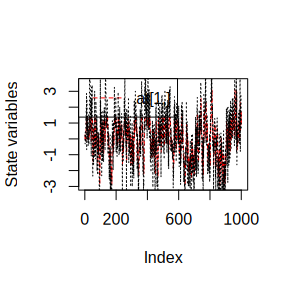and comparision of the filtered series with the realization

plot(ans, at.idx = NA, att.idx = 1, CI = NA)
lines(a, lty = "dotted")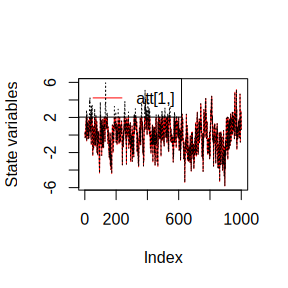The plotting function for the fkf class also allows visual checking of whether the residuals are Gaussian

plot(ans, type = "resid.qq")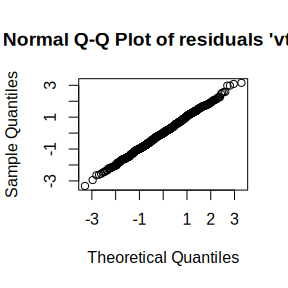or if there is a linear serial dependence through ‘acf’

plot(ans, type = "acf")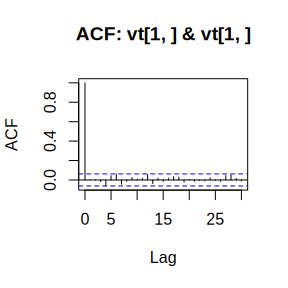The smoothed estimates can also be computed by a simple call to fks

sm <- fks(ans)

The smoothed estiamte with confidence intervals can be plotted alongside the observed data

plot(sm)
lines(a,col="black", lty="dotted")